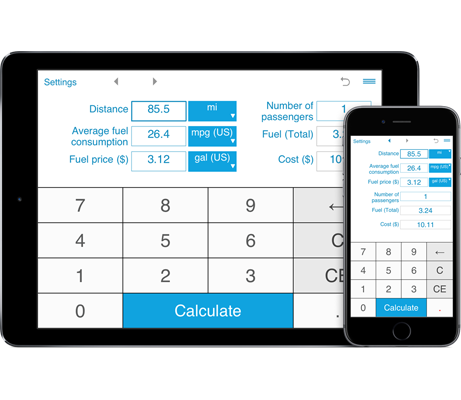# Fuel cost calculator

Need to calculate how much petrol or diesel you need for your trip? Not sure if it can be more cost efficient to take a plane instead? This handy fuel cost calculator lets you easily and quickly estimate the fuel consumption and fuel cost of a trip.
If you have any passengers in your car, you can enter the number of passengers and the calculator will calculate you the cost of your trip per passenger so you can divide the total fuel cost of your trip amongst everyone who is travelling with you.

## Calculate the fuel cost for your trip

The fuel trip cost calculator allows you to calculate how much fuel you need for your trip and how much money it will cost.
The trip fuel cost calculator performs calculations in US, imperial and metric units.
The calculator will be especially useful when driving a longer distance as it helps you decide whether the trip fuel cost is worth the travel by car. Simply enter the details of your trip and the calculator will show you how much fuel your car is predicted to consume (Fuel Total) and how much a trip will cost (Total Cost).

Enter the distance (in miles or kilometers), the average fuel consumption for your car (in MPG US, MPG UK, km/L or L/100 km) and the fuel price and the calculator calculates the gas cost or diesel cost of your trip.

The fuel calculator keeps calculation history so you can easily view and compare your recent calculations to choose the most cost efficient solution.

You can easily share the results of your calculations via email.